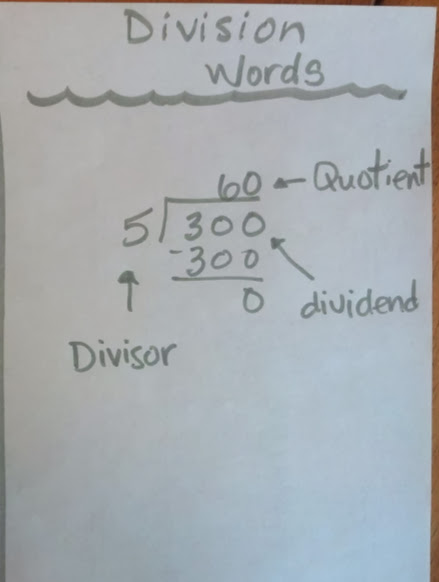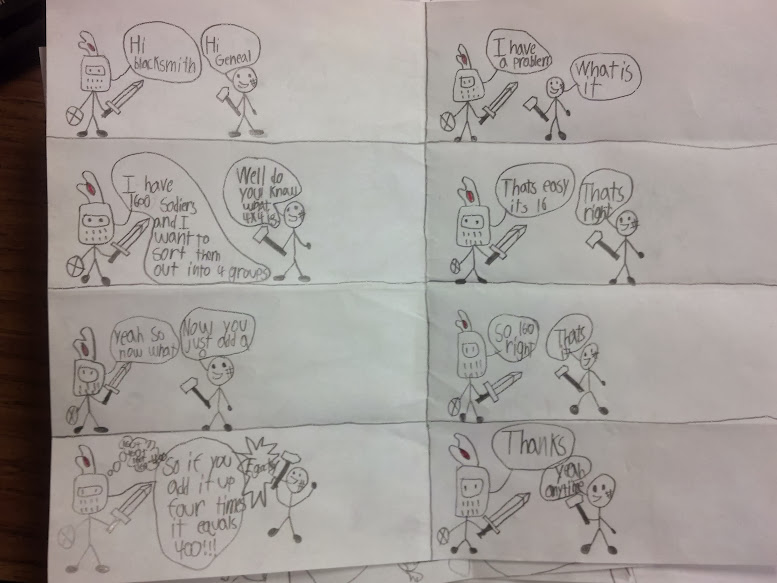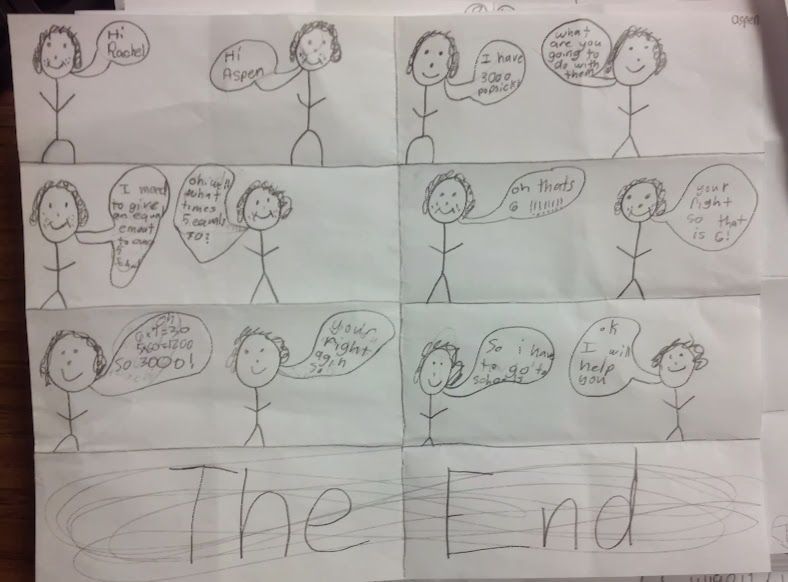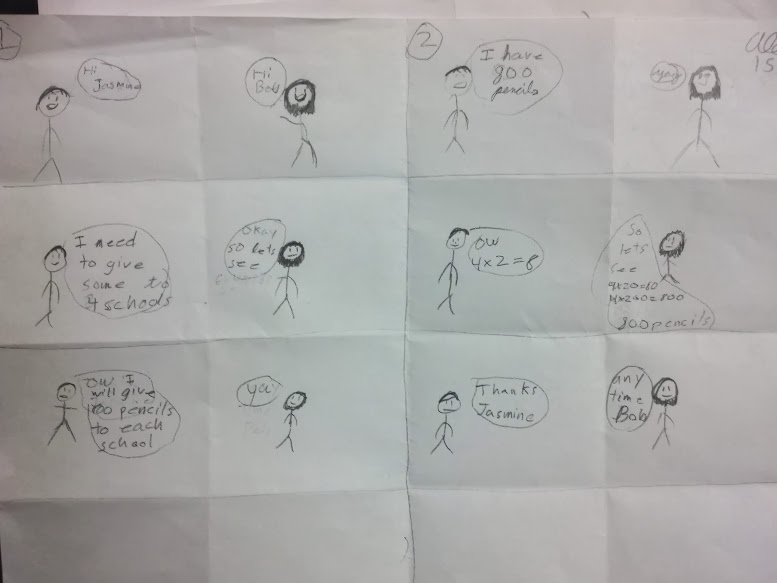# Lots of Laughs with Finding Unknown Factors

2 teachers like this lesson
Print Lesson

## Objective

SWBAT report the quotient of a division problem as a whole number and discover relationships between dividing tens, hundreds, and thousands.

#### Big Idea

Ha ha ha! LOL! Students create comic strips in this lesson in order to solidify academic vocabulary of division while dividing multiples of ten.

## Warm Up

8 minutes

Students will start today's lesson with a fluency assessment.  This assessment is from Monitoring Basic Skills Progress Second Edition: Basic Math Computation by Lynn S. Fuchs, Carol L. Hamlett, and Douglas Fuchs.

This is an assessment I have my students do each week and then graph their results.  It allows them to reflect on their learning of basic math facts, as well as using all four operations with whole numbers, and adding and subtracting unit fractions.  (It also happens to be the quietest time in my math classroom all week!!)

This is what my classroom looks like as students work on this assessment.

This is a sample of a student's graph.  Please check out the resources to hear my thoughts about students fluency progress.   Since students began the fourth grade set today, I anticipate students graphs to decrease in the number corrected and attempted.

Students began the fourth grade set this week.  I do not start my students with the fourth grade skills. I chose to start them with the end of the third grade skills which covers addition, subtraction and multiplication and division of basic facts. I strongly believe in a balanced math approach, which is one reason why I also believe in common core standards.  By having a balance of building conceptual understanding, application of problems, and computational fluency, students can experience rigorous mathematics.  I want to make clear that this assessment ONLY measures basic math computation.  It is only one piece of students' knowledge. The assessments in this book, for each grade level, do not change in difficulty over the course of the year.  Therefore, a student's increase in score over the school year truly reflects improvement in the student's ability to work the math problems at that grade level.

## Concept Development

50 minutes

Note: This lesson was written for a guest teacher.  While the text in this narrative says "I", a guest teacher carried out this lesson with ease, leaving me a note that students enjoyed the lesson and that she wished she was in fourth grade math. Upon returning to school, my students did demonstrate a solid understanding of being able to use the terms dividend, divisor, and quotient.

I write on the board 30 ÷ 5 and 300 ÷ 5.   I ask students what these expressions mean and if they can come up with a story to go with each expression. After students respond, I write the related multiplication facts below the division expressions.

Next, I have students take notes about division in their math notebooks. Some things they take notes on are: what is division, what is a quotient, divisor, and dividend. I then ask students to discuss what the relationship between multiplication and division are.

You can see in this photo one way I have students label the parts of a division problem in their math notebooks.After finishing their notes, students work in table groups to manipulate larger division problems. I give each group a bag of ones blocks, a bag of tens blocks, and a bag of hundreds blocks. I start out by having students show a division problem with the ones blocks like 27/3=9, and then have them show 270/3 with tens, and 2700/3 with hundreds. Then I see if students can use the patterns to determine 27,000/3.

Students make 4 four column T-chart in notebooks, where they label columns ones, tens, hundreds, and thousands. I give them a problem in the ones, and have them use that problem to find the quotients in the next place value up with and without place value blocks.

Next I give them real world problems like: If I have 300 books and I need to put an equal amount on 6 shelves, how many would go on each shelf? I have the students use place value patterns to solve problems like these.

For the remainder of the lesson, students will make a comic strip displaying a division problem.  Students are encouraged to use a number in the thousands in their comic strip. On the back of their comic strip, I ask students to write about the related division fact that helped them determine the quotient for the larger division problem.

These photos are samples of student comic strips.This activity incorporates Math Practice Standards 7 and 8.  In order to identify the structure of a mathematical problem, students often need to engage in some form of visual learning or visualization. When students make connections between the patterns of multiplication and adding zeros to the product equal to the sum of the zeros in the factors, and realize this is also true for division, they use a pattern previously discovered.